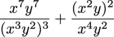# TSI Math: Simplifying Fractions with a Ton of Exponents

Simplify the following expression.Intermediate Algebra and Functions Rational and Exponential Expressions, Equations, and Functions Mathematics and Statistics Assessment Rational and Exponential Expressions, Equations, and Functions Product Type TSI TSI Mathematics and Statistics AssessmentTSI MathTSI Mathematics TSI Math Intermediate Algebra and Functions TSI Mathematics Intermediate Algebra and Functions Test Prep TSI

### Transcript

00:20

power or experiment number there Yeah well the extra exponents

00:23

are knocked out by the power to a power rule

00:26

right When exponential expressions are raised to a power we

00:30

can multiply the exponents together That'll help us a whole

00:34

lot here All right so we're going to do some

00:36

00:39

So we've got x word y to the second power

00:41

which is the same as x squared times two and

00:44

then times y to the one times two thinking there

00:47

and then on the bottom here we got this thing

00:49

cubed that's going to be the same as x to

00:52

the three times three And why did the two times

00:54

three and let's just go do all the arithmetic here

00:57

So we got xs seventh wanted seventh over x to

00:59

the ninth one of the six and all this stuff

01:01

Okay bam How the expression is starting to shrink Hey

01:05

it's good Use that x ray vision to identify expressions

01:07

that cancel in the first term of six wise in

01:10

the denominator cancel with six of the seven wise in

01:13

the numerator leaving just one behind you is gonna look

01:16

like this Why right there why over x squared Is

01:19

it The seven x is in the numerator Cancel seven

01:22

of the nine x is in the denominator leading just

01:25

two exes They're cease oh that's way simpler Why over

01:28

x squared that's What All this stuff up here reduced

01:30

down teo Yeah so it's kind of good All right

01:33

so let's keep going Now the second fraction this one

01:35

is identical in the numerator and denominator so the entire

01:39

fractions just one that's it it's Why over x squared

01:42

plus one after all that work Yep Are super human

01:46

ability to use exponents rule to fight evil one simplified

01:49

expressions leaves us to truth justice and the shmoop way 00:01:52.663 --> [endTime] Yeah so why over x y plus one